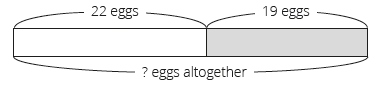# Lesson Notes By Weeks and Term - Primary 2

TERM: 2nd Term

WEEK: 2

CLASS: Primary 2

AGE: 7 years

DURATION: 5 periods of 40 minutes each

DATE:

SUBJECT: Mathematics

SPECIFIC OBJECTIVES: At the end of the lesson, the pupils should be able to

1. Practise adding and subtracting 2-digit numbers using the column method.
2. Solve the column method of addition with carrying in the ones place using a base ten kit.
3. Practise addition of 2-digit numbers with carrying using the column method. Use commutative property to check the answer.
4. Solve addition problems with carrying on a number line.

INSTRUCTIONAL TECHNIQUES: Explanation, question and answer, demonstration, practical

INSTRUCTIONAL MATERIALS: Printed tens, bottle tops and place value table per learner,

PERIOD 1: Assessment

 PRESENTATION TEACHER’S ACTIVITY PUPIL’S ACTIVITY STEP 1ORALASSESSMENTS The teacher asks questions on mental maths treated so far Pupils respond and participate STEP 2DISCUSSION The teacher discusses all the methods used by some learners in the oral assessments(some of the questions are solved on the board by the learners) and addresses any misconceptions that may have risen Pupils pay attention and participate STEP 3WRITTEN ASSESSMENTS 1 Solve 27 + 35 = ___  using a base ten kit and place value table.2. Solve 27 + 35 = ___ using a number line.3. Calculate 27 + 35 = ___ using the column method.4 Circle the method that you found easiest.(Learners circle one of their solutions above. There is not wrong answer here; they might find any of the methods easiest. Ask them to explain their answers verbally if there is time. Answers will vary. E.g. I found the column method easiest because it isquick/ I understand it/ I don’t need to break down 35 into 3, 30 and 2. OR I found the number line method is easiest, because I need less space to work on it, etc. Learners do not have to write the explanation here.) Pupils attempt their class work STEP 4SUMMARY The teacher marks the written assessments, corrects were necessary and commends the pupils

PERIOD 2: Addition with carrying in context

 PRESENTATION TEACHER’S ACTIVITY PUPIL’S ACTIVITY STEP 1MENTAL MATHS The teacher begins the lesson with some mental calculationsCalculate1 8 + 3 =   2 9 + 5 =    3 6 + 9 =    4 7 + 7 =    5 9 + 8 =    6 7 + 5 =7 8 + 3 =8 6 + 8 =9 7 + 8 =10 9 + 9 = Pupils respond and participate STEP 2CONCEPTDEVELOPMENT The teacher• Writes the following word problem on the board.• This is an example of an addition (combine) question.Nosa has 34 green marbles and28 blue marbles.How many marbles does Nosa have altogether?The word problem must be written as above (3 separate lines) to assist learners to identify the critical information/numbers needed to solve the problem.• Reads the problem.• Lets learners read the problem until they read it fluently.• Underlines the numbers, 34 and 28.• Underlines the question (How many marbles does Nosisi have altogether?) with a wavy line.• Draws the following bar diagram.• Lets learners copy the diagram into their classwork books.• Lets learners write the number sentence.• Lets learners present their number sentence and explain which operation should be used to calculate the solution to the problem.• Lets the learners solve the number sentence (34 + 28 = 62).• Asks: What is the answer for the word problem? (Nosa has 62 marbles).• Learners have to answer with the unit, 62 marbles. CLASS ACTIVITYThe teacher• This second activity is an example of addition (compare).• Let learners solve the problem below by following the steps in Activity 1.My mom is 32 years old andmy dad is 9 years older than her.How old is my dad?• The bar diagram for this problem is as follows. Draw it on the board for the learners to copy.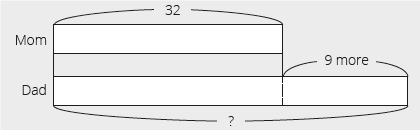• The number sentence that expresses the solution is 32 + 9 = __ (41).• The answer is my dad is 41 years old. Pupils pay attention and participate STEP 3CLASS-WORK This classwork should be done as a class so that you and the learners can discuss and solve the problems together. Write the question and draw a bar diagram. Let learners find the number sentence that expresses the solution to the problem and solve it. You must write the problems on the board (in 3 lines, to show the information clearly) and draw the diagrams with the learners. They will not draw the diagrams by themselves in this lesson (they will do this in a later lesson). The first example below is addition combine and the second one is addition compare. Solve the following problems:1 I had 23 oranges.My dad gave me 9 oranges.How many do I have now?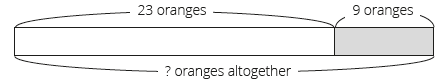2. Bayo has 27 bananas.Silas has 8 more bananas than Bayo.How many bananas does Silas have?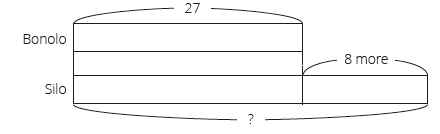Pupils attempt their class work STEP 4HOME-WORK 1 Thanos has 47 green crayons and5 yellow crayons.How many crayons does Thanos have altogether?The pupils writes it in their homework book STEP 5SUMMARY The teacher summarizes by reminding the pupils that in this lesson we solved real life addition problems with carrying. We used bar diagrams to solve these problems. She marks their class works, makes corrections where necessary and commends them positively

PERIOD 3: Making addition number sentences

PERIOD 4: Assessment

 PRESENTATION TEACHER’S ACTIVITY PUPIL’S ACTIVITY STEP 1ORALASSESSMENTS The teacher asks questions on mental maths treated so far Pupils respond and participate STEP 2DISCUSSION The teacher discusses all the methods used by some learners in the oral assessments(some of the questions are solved on the board by the learners) and addresses any misconceptions that may have risen Pupils pay attention and participate STEP 3WRITTEN ASSESSMENTS Solve the following using the bar diagrams. Write a number sentence to show your answer.1 Stanley has 43 yellow marblesand 28 black marbles.How many marbles does Stanley have?2 Jack has 27 sweets.Jill has 8 more than Jack.How many sweets does Jill have?3. Nene has 36 apples.Her mom gave her 8 apples.How many apples does she have now?4 Use the column method to check these answers. Mark each one with a tick or a cross.a 22 + 27 = 49b 39 + 58 = 100 Pupils attempt their class work STEP 4SUMMARY The teacher marks the written assessments, corrects were necessary and commends the pupils

PERIOD 5: Weekly Test/consolidations

TEACHER’S ACTIVITY: The teacher revises all the concepts treated from period 1-4 and gives the pupils follow through exercises, quiz and tests. She marks the exercises, makes corrections and commends the pupils positively.

PUPIL’S ACTIVITY: The pupils work on the worksheets and exercises give by the teacher individually

CONSOLIDATION

1 Use the column method to check this answer. Mark it with a tick or a cross.

46 + 19 = 65

1. Solve using a bar diagram. Write a number sentence to show your answer.

a Zeta has 33 red pencils and

17 blue pencils.

How many pencils does Zeta have?b Jack is 25 years old.

James is 8 years older.

How old is James?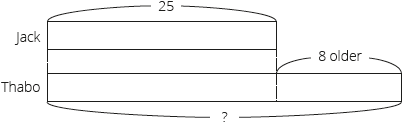c Boma has 22 eggs.

He mom gave her 19 more eggs.

How many eggs does she have now?